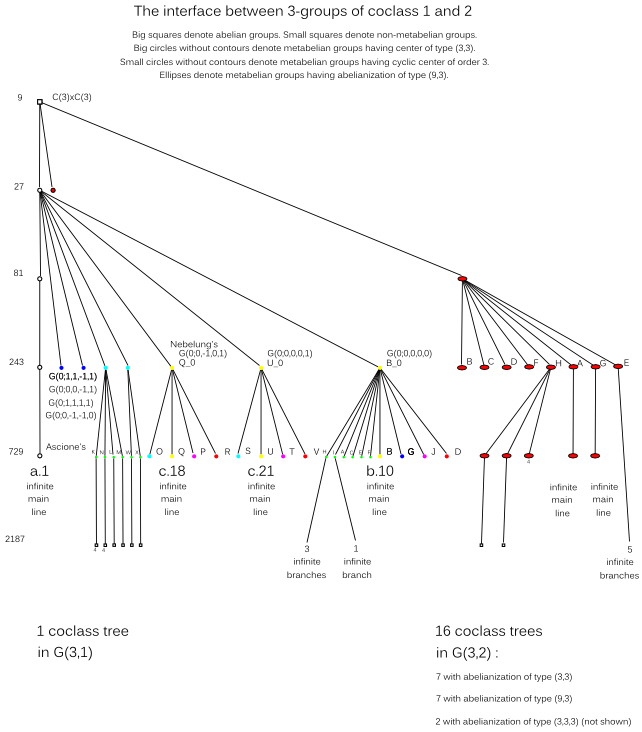# Oujda Research Project 2011

## Interface between the Coclass Graphs G(3,1) and G(3,2)

The vertices of the coclass graph G(3,2) represent all finite 3-groups G of coclass cc(G)=2.
G(3,2) consists of finitely many sporadic groups and 16 coclass trees,
each with a single infinite main line and a structural periodicity.

The interface between G(3,1) and G(3,2) consists of connecting
two groups G ∈ G(3,1) and H ∈ G(3,2) by a directed edge of depth two, G→H,
if G = H/N, where N denotes the last non-trivial member of the lower central series of H,
in the case that N is bicyclic of type (3,3).
Exceptionally, the directed edges of depth two connect two non-adjacent horizontal planes
with constant orders 3n and 3n+2, differing by a factor 9.

Only the top of 5 coclass trees with metabelian main lines is shown here.
For the detailed structural periodicity see the following links:Applications in class field theory:
The sporadic vertices of G(3,2), which are located outside of infinite coclass trees,
are particularly favored as second 3-class groups of quadratic number fields K = Q(sqrt(D)),
that is, Galois groups Gal(F32(K)|K) of the second Hilbert 3-class field.
They are either completely isolated or located at depth one on finite trees.

Among the 2020 complex quadratic fields with discriminant -106 < D < 0 having a 3-class group of type (3,3),
we have 1888 (93.5%) cases with second 3-class groups on G(3,2).
For these, the dominating part of 1327 (70.3%) has a sporadic second 3-class group.
We have 667 hits of type D.10 (2241) and 269 hits of type D.5 (4224), both isolated of order 243,
297 hits of type H.4 (4443) and 94 hits of type G.19 (2143), both of order 729 at depth one on finite trees.

Among the 2576 real quadratic fields with discriminant 0 < D < 107 having a 3-class group of type (3,3),
only a minor part of 260 (10.1%) has second 3-class groups on G(3,2).
For these, the dominating part of 178 (68.5%) has a sporadic second 3-class group.
We have 93 hits of type D.10 (2241) and 47 hits of type D.5 (4224), both isolated of order 243,
27 hits of type H.4 (4443) and 11 hits of type G.19 (2143), both of order 729 at depth one on finite trees.

 * Web master's e-mail address: contact@algebra.at *